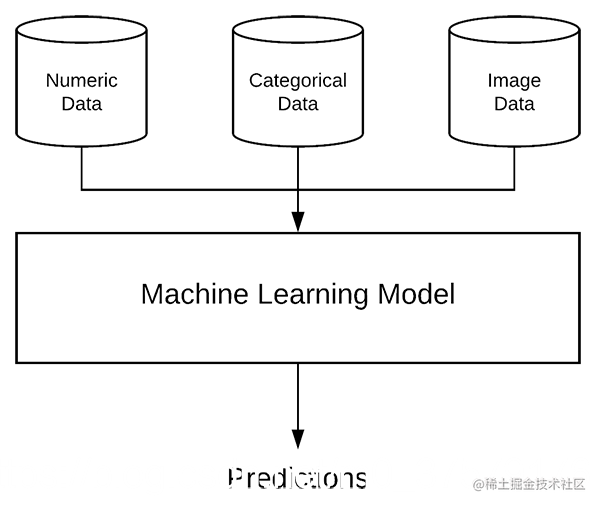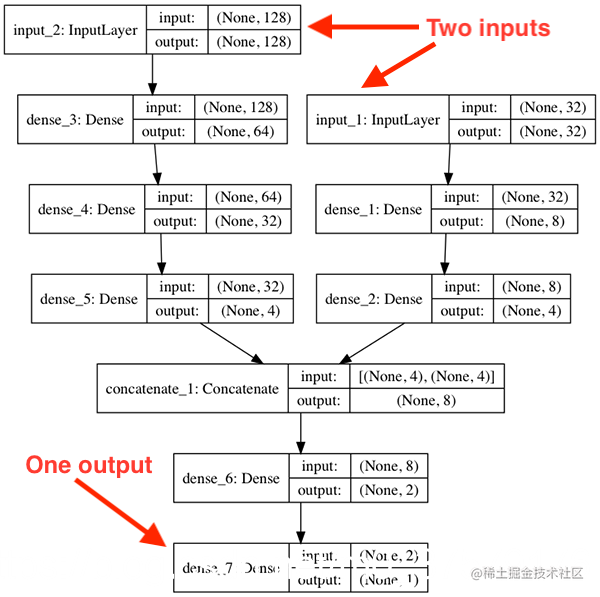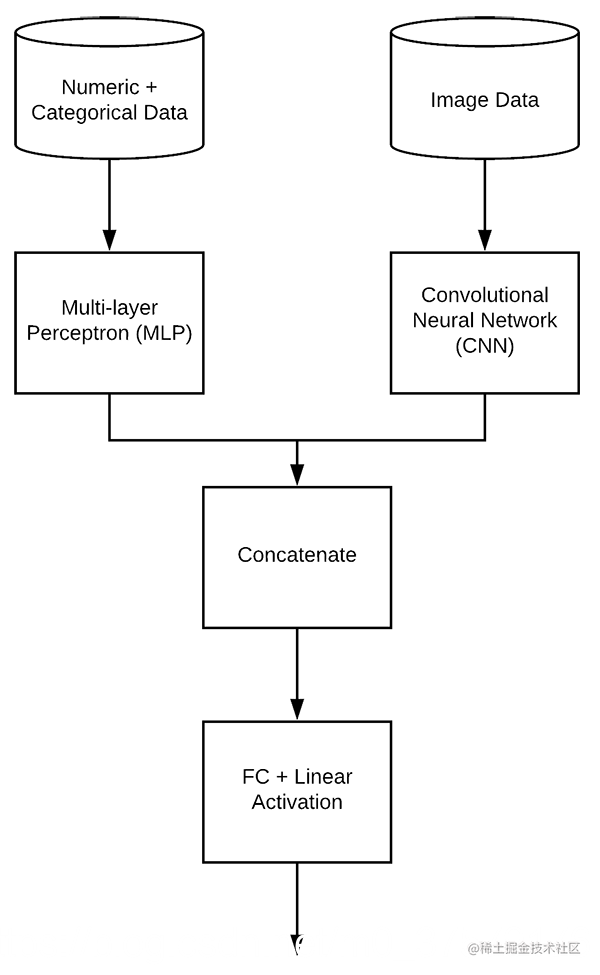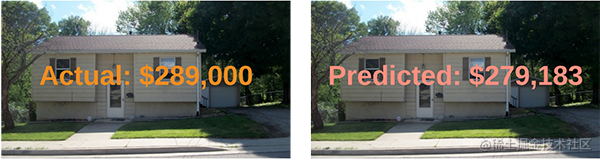Keras如何接受多个输入?

摘要

1. 定义一个Keras模型，该模型能够同时接受多个类型的输入数据，包括数值、类别和图像等类型的数据。
2. 在混合数据输入上训练端到端的Keras模型。
3. 使用多个输入来评估我们的模型。

正文

什么是混合数据?1. 数值/连续值，如年龄、心率、血压
2. 类别值，包括性别和种族
3. 图像数据，如MRI、x片等。

Keras如何接受多个输入?

Keras能够通过它的函数API处理多个输入(甚至多个输出)。

• 多输入模型
• 多输出模型
• 模型包括多个输入和多个输出
• 有向无环图
• 具有共享层的模型

model = Sequential()

inputs = Input(shape=(10,))
x = Dense(8, activation="relu")(inputs)
x = Dense(4, activation="relu")(x)
x = Dense(1, activation="linear")(x)
model = Model(inputs, x)

# define two sets of inputs
inputA = Input(shape=(32,))
inputB = Input(shape=(128,))

# the first branch operates on the first input
x = Dense(8, activation="relu")(inputA)
x = Dense(4, activation="relu")(x)
x = Model(inputs=inputA, outputs=x)

# the second branch opreates on the second input
y = Dense(64, activation="relu")(inputB)
y = Dense(32, activation="relu")(y)
y = Dense(4, activation="relu")(y)
y = Model(inputs=inputB, outputs=y)

# combine the output of the two branches
combined = concatenate([x.output, y.output])

# apply a FC layer and then a regression prediction on the
# combined outputs
z = Dense(2, activation="relu")(combined)
z = Dense(1, activation="linear")(z)

# our model will accept the inputs of the two branches and
# then output a single value
model = Model(inputs=[x.input, y.input], outputs=z)

• inputA: 32维
• inputB: 128维房价数据集1. 数量的卧室
2. 数量的浴室
3. 区域(面积)
4. 邮政编码

1. 卧室
2. 浴室
3. 厨房
4. 房子的正面

项目结构

\$ tree --dirsfirst --filelimit 10
.
├── Houses-dataset
│   ├── Houses\ Dataset [2141 entries]
├── pyimagesearch
│   ├── __init__.py
│   ├── datasets.py
│   └── models.py
└── mixed_training.py

3 directories, 5 files

Houses-dataset文件夹包含我们在本系列中使用的房价数据集。当我们准备好运行mixed_training.py脚本时，您只需要提供一个路径作为数据集的命令行参数(我将在结果部分向您详细说明这是如何完成的)。

• pyimagesearch/datasets.py: 加载和预处理我们的数字数据，类别数据以及图像数据。
• pyimagesearch/models.py: 包含多层感知器(MLP)和卷积神经网络(CNN)。这些组件是我们的多输入混合数据模型的输入分支。
• mixed_training.py: 首先我们的训练脚本将使用pyimagesearch模块来加载和分割训练数据集，添加数据头，并将两个分支连接到我们的网络。然后对模型进行培训和评估。

加载数值和分类数据

# import the necessary packages
from sklearn.preprocessing import LabelBinarizer
from sklearn.preprocessing import MinMaxScaler
import pandas as pd
import numpy as np
import glob
import cv2
import os

# initialize the list of column names in the CSV file and then
cols = ["bedrooms", "bathrooms", "area", "zipcode", "price"]

# determine (1) the unique zip codes and (2) the number of data
# points with each zip code
zipcodes = df["zipcode"].value_counts().keys().tolist()
counts = df["zipcode"].value_counts().tolist()

# loop over each of the unique zip codes and their corresponding
# count
for (zipcode, count) in zip(zipcodes, counts):
# the zip code counts for our housing dataset is *extremely*
# unbalanced (some only having 1 or 2 houses per zip code)
# so let's sanitize our data by removing any houses with less
# than 25 houses per zip code
if count < 25:
idxs = df[df["zipcode"] == zipcode].index
df.drop(idxs, inplace=True)

# return the data frame
return df

def process_house_attributes(df, train, test):
# initialize the column names of the continuous data
continuous = ["bedrooms", "bathrooms", "area"]

# performin min-max scaling each continuous feature column to
# the range [0, 1]
cs = MinMaxScaler()
trainContinuous = cs.fit_transform(train[continuous])
testContinuous = cs.transform(test[continuous])

# one-hot encode the zip code categorical data (by definition of
# one-hot encoding, all output features are now in the range [0, 1])
zipBinarizer = LabelBinarizer().fit(df["zipcode"])
trainCategorical = zipBinarizer.transform(train["zipcode"])
testCategorical = zipBinarizer.transform(test["zipcode"])

# construct our training and testing data points by concatenating
# the categorical features with the continuous features
trainX = np.hstack([trainCategorical, trainContinuous])
testX = np.hstack([testCategorical, testContinuous])

# return the concatenated training and testing data
return (trainX, testX)

加载图像数据集# initialize our images array (i.e., the house images themselves)
images = []

# loop over the indexes of the houses
for i in df.index.values:
# find the four images for the house and sort the file paths,
# ensuring the four are always in the *same order*
basePath = os.path.sep.join([inputPath, "{}_*".format(i + 1)])
housePaths = sorted(list(glob.glob(basePath)))

1. 从房价数据集中加载所有照片。回想一下，我们每个房子有四张照片(图6)。
2. 从四张照片生成一个单一的拼合图像。拼合图像总是按照你在图中看到的那样顺序排列。
3. 将所有这些主蒙版添加到列表/数组中并返回到调用函数。

• 初始化图像列表(第61行)并将用我们创建的所有拼合图像填充这个列表。
• 循环遍历数据帧中的房屋(第64行)以获取当前住宅的四张照片的路径

# initialize our list of input images along with the output image
# after *combining* the four input images
inputImages = []
outputImage = np.zeros((64, 64, 3), dtype="uint8")

# loop over the input house paths
for housePath in housePaths:
# load the input image, resize it to be 32 32, and then
# update the list of input images
image = cv2.resize(image, (32, 32))
inputImages.append(image)

# tile the four input images in the output image such the first
# image goes in the top-right corner, the second image in the
# top-left corner, the third image in the bottom-right corner,
# and the final image in the bottom-left corner
outputImage[0:32, 0:32] = inputImages
outputImage[0:32, 32:64] = inputImages
outputImage[32:64, 32:64] = inputImages
outputImage[32:64, 0:32] = inputImages

# add the tiled image to our set of images the network will be
# trained on
images.append(outputImage)

# return our set of images
return np.array(images)

• 在循环中，我们:

• 执行初始化(第72行和第73行)。我们的inputImages将以列表的形式包含每条记录的四张照片。我们的inputImages将是照片的拼接图像(如图6所示)。

• 循环4张照片(第76行):

• 加载、调整大小并将每张照片附加到 inputImages中(第79-81行)。
• 为四个房子的图片(第87-90行)创建平铺(拼接图像):

• 左上方的浴室图片。
• 右上角的卧室图片。
• 右下角的正面视图。
• 厨房在左下角。
• 添加拼接outputImageimages(第94行)。

• 跳出循环，我们以NumPy数组的形式返回所有图像(第97行)。

定义多层感知器(MLP)和卷积神经网络(CNN)• 数字和分类数据
• 图像数据

• 第一个分支是一个简单的多层感知器(MLP)，用于处理数值输入。
• 第二个分支是卷积神经网络，用于对图像数据进行操作。
• 然后将这些分支连接在一起，形成最终的多输入Keras模型。

# import the necessary packages
from keras.models import Sequential
from keras.layers.normalization import BatchNormalization
from keras.layers.convolutional import Conv2D
from keras.layers.convolutional import MaxPooling2D
from keras.layers.core import Activation
from keras.layers.core import Dropout
from keras.layers.core import Dense
from keras.layers import Flatten
from keras.layers import Input
from keras.models import Model

def create_mlp(dim, regress=False):
# define our MLP network
model = Sequential()

# check to see if the regression node should be added
if regress:

# return our model
return model

MLP由create_mlp定义。

• 具有ReLU激活的完全连接(密集)输入层
• 一个完全连接的隐藏层，也带有ReLU激活
• 最后，一个线性激活的可选的回归输出

def create_cnn(width, height, depth, filters=(16, 32, 64), regress=False):
# initialize the input shape and channel dimension, assuming
# TensorFlow/channels-last ordering
inputShape = (height, width, depth)
chanDim = -1

# define the model input
inputs = Input(shape=inputShape)

# loop over the number of filters
for (i, f) in enumerate(filters):
# if this is the first CONV layer then set the input
# appropriately
if i == 0:
x = inputs

# CONV => RELU => BN => POOL
x = Conv2D(f, (3, 3), padding="same")(x)
x = Activation("relu")(x)
x = BatchNormalization(axis=chanDim)(x)
x = MaxPooling2D(pool_size=(2, 2))(x)

create_cnn函数处理图像数据并接受五个参数:

• 宽度:输入图像的宽度，单位为像素。
• 高度:输入图像的高度，单位为像素。
• 深度:输入图像中的通道数。对于RGB彩色图像，它是3。
• 过滤器:一组逐渐变大的过滤器，使我们的网络可以学习更多的区分功能。
• 回归:一个布尔值，指示是否将一个完全连接的线性激活层添加到CNN以进行回归。

# flatten the volume, then FC => RELU => BN => DROPOUT
x = Flatten()(x)
x = Dense(16)(x)
x = Activation("relu")(x)
x = BatchNormalization(axis=chanDim)(x)
x = Dropout(0.5)(x)

# apply another FC layer, this one to match the number of nodes
# coming out of the MLP
x = Dense(4)(x)
x = Activation("relu")(x)

# check to see if the regression node should be added
if regress:
x = Dense(1, activation="linear")(x)

# construct the CNN
model = Model(inputs, x)

# return the CNN
return model

使用Keras的多个输入

# import the necessary packages
from pyimagesearch import datasets
from pyimagesearch import models
from sklearn.model_selection import train_test_split
from keras.layers.core import Dense
from keras.models import Model
from keras.layers import concatenate
import numpy as np
import argparse
import locale
import os

# construct the argument parser and parse the arguments
ap = argparse.ArgumentParser()
help="path to input dataset of house images")
args = vars(ap.parse_args())

• datasets: 我们的三个方便的功能，从房屋数据集加载/处理CSV数据和加载/预处理房屋照片。
• models: 我们的MLP和CNN输入分支,它们将作为我们的多输入混合数据服务。
• train_test_split: 一个scikit-learn函数，用于构造我们的训练/测试数据分割。
• concatenate: 一个特殊的Keras函数，它将接受多个输入。
• argparse: 处理解析命令行参数。

# construct the path to the input .txt file that contains information
# on each house in the dataset and then load the dataset
inputPath = os.path.sep.join([args["dataset"], "HousesInfo.txt"])

# load the house images and then scale the pixel intensities to the
# range [0, 1]
images = images / 255.0

# partition the data into training and testing splits using 75% of
# the data for training and the remaining 25% for testing
print("[INFO] processing data...")
split = train_test_split(df, images, test_size=0.25, random_state=42)
(trainAttrX, testAttrX, trainImagesX, testImagesX) = split

# find the largest house price in the training set and use it to
# scale our house prices to the range [0, 1] (will lead to better
# training and convergence)
maxPrice = trainAttrX["price"].max()
trainY = trainAttrX["price"] / maxPrice
testY = testAttrX["price"] / maxPrice

# process the house attributes data by performing min-max scaling
# on continuous features, one-hot encoding on categorical features,
# and then finally concatenating them together
(trainAttrX, testAttrX) = datasets.process_house_attributes(df,
trainAttrX, testAttrX)

process_house_attributes函数处理这些操作，并将连续的和分类的特性连接在一起，返回结果(第48行和第49行)。

# create the MLP and CNN models
mlp = models.create_mlp(trainAttrX.shape, regress=False)
cnn = models.create_cnn(64, 64, 3, regress=False)

# create the input to our final set of layers as the *output* of both
# the MLP and CNN
combinedInput = concatenate([mlp.output, cnn.output])

# our final FC layer head will have two dense layers, the final one
x = Dense(4, activation="relu")(combinedInput)
x = Dense(1, activation="linear")(x)

# our final model will accept categorical/numerical data on the MLP
# input and images on the CNN input, outputting a single value (the
# predicted price of the house)
model = Model(inputs=[mlp.input, cnn.input], outputs=x)

# compile the model using mean absolute percentage error as our loss,
# implying that we seek to minimize the absolute percentage difference
# between our price *predictions* and the *actual prices*
opt = Adam(lr=1e-3, decay=1e-3 / 200)
model.compile(loss="mean_absolute_percentage_error", optimizer=opt)

# train the model
print("[INFO] training model...")
model.fit(
[trainAttrX, trainImagesX], trainY,
validation_data=([testAttrX, testImagesX], testY),
epochs=200, batch_size=8)

# make predictions on the testing data
print("[INFO] predicting house prices...")
preds = model.predict([testAttrX, testImagesX])

# compute the difference between the *predicted* house prices and the
# *actual* house prices, then compute the percentage difference and
# the absolute percentage difference
diff = preds.flatten() - testY
percentDiff = (diff / testY) * 100
absPercentDiff = np.abs(percentDiff)

# compute the mean and standard deviation of the absolute percentage
# difference
mean = np.mean(absPercentDiff)
std = np.std(absPercentDiff)

# finally, show some statistics on our model
locale.setlocale(locale.LC_ALL, "en_US.UTF-8")
print("[INFO] avg. house price: {}, std house price: {}".format(
locale.currency(df["price"].mean(), grouping=True),
locale.currency(df["price"].std(), grouping=True)))
print("[INFO] mean: {:.2f}%, std: {:.2f}%".format(mean, std))

多输入和混合数据结果1. 根据本系列的第一个教程配置开发环境。
2. 使用本教程的源代码。
3. 使用上面“获取房价数据集”一节中的说明下载房价数据集。

\$ python mixed_training.py --dataset Houses-dataset/Houses\ Dataset/

1. 仅对数值/分类数据使用MLP: 26.01%
2. 仅用CNN对图像数据:56.91%
3. 使用混合数据：22.41%

1. 结合我们的数字/l类别数据和图像数据
2. 对混合数据进行多输入模型的训练。
3. 带来了一个性能更好的模型!

• 数值数据
• 分类数据
• 图像数据